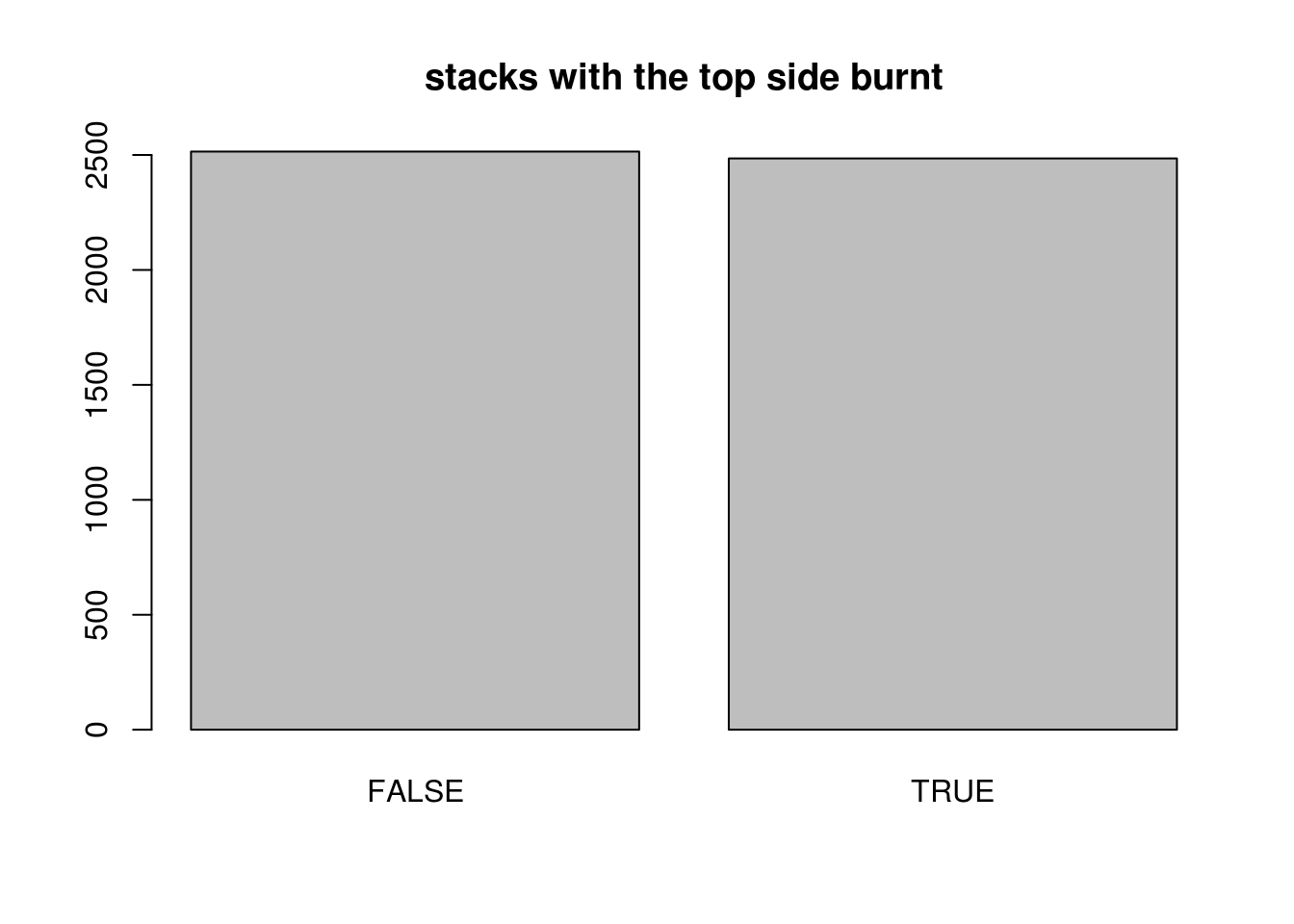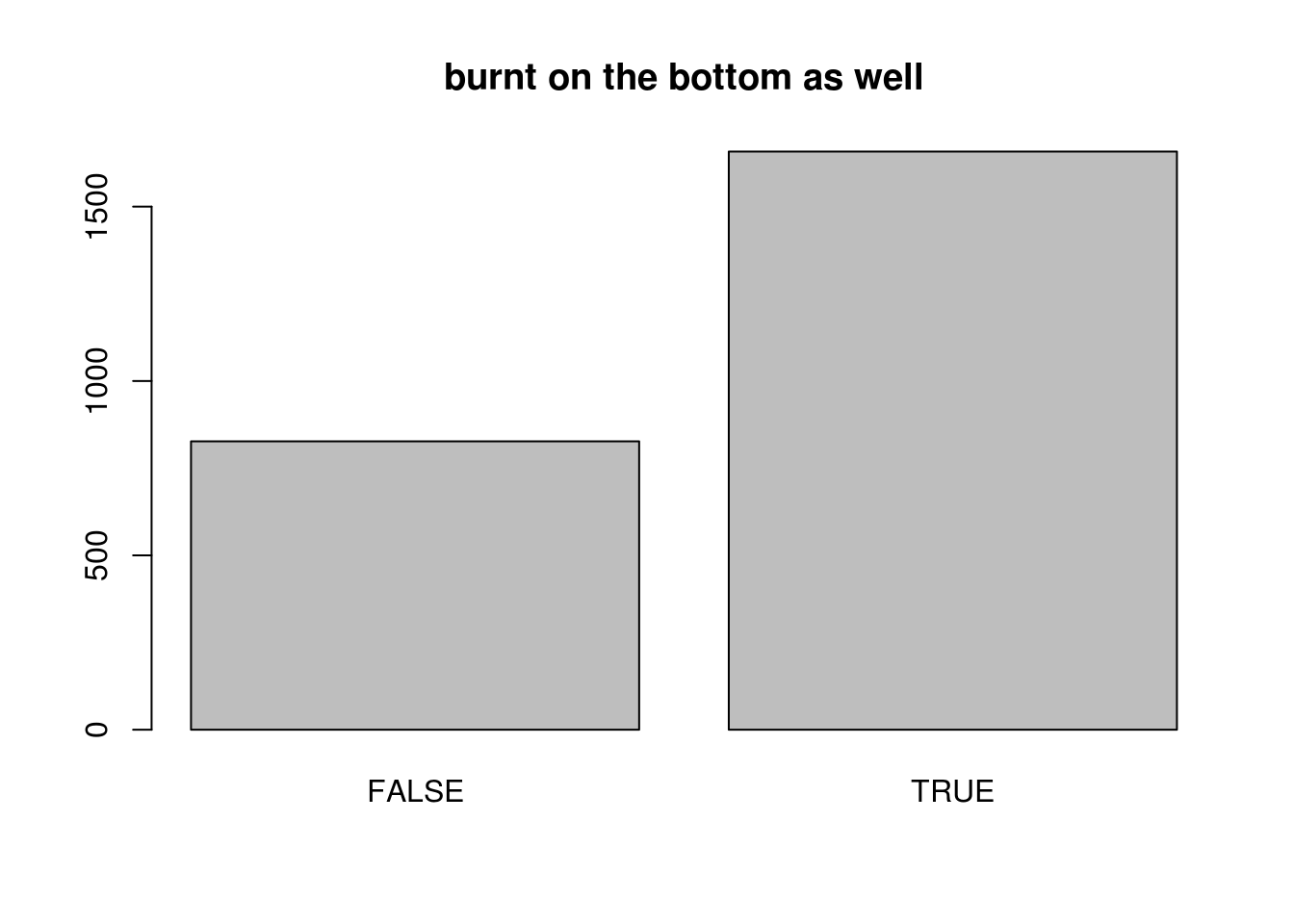## Intoduction

The Pancake Problem (which is sometimes stated using coloured cards instead of pancakes) provides an example of a probability problem that is easy to state and understand (well, at least we think we understand it), and yet yeilds contentious results.

Your friend is cooking you some pancakes, and you see that they have had some mixed results: one pancake is nice and golden brown on both sides, one is burnt on one side and brown on the other, and the third is burnt on both sides. When the stack of pancakes is presented to you, the exposed side of the top pancake is burnt. What is the probability that the top pancake is the one that is burnt on both sides?

Well, let’s see how likely it is that the top pancake is twice-burnt by writing a simulation.

## Setting up the Simulation

First weset up a Pancake dataframe with 5000 trials.

trial_limit <- 5000
trial <- 1:trial_limit
Pancake <- data.frame(trial)

Now, we will cookup a batch of pankakes matching the description in the problem: one not burnt, one burnt on one side, one burnt on both sides. For each side of each cake we will answer the question “is this side burnt?” with a boolean. The pancakes then go into a list that we will sample.

not_burnt <- c(FALSE,FALSE )
half_burnt <- c(TRUE, FALSE)
all_burnt <- c(TRUE, TRUE)
cakes <- list(not_burnt, half_burnt, all_burnt)

Each trial is a possible stack of these pancakes. First we will randomly select a pancake to put on top, then randomly pick a side to show face up.

Pancake$topCake <- sample(cakes, trial_limit, replace=TRUE) for (i in trial) { side <- sample(1:2,1) Pancake$topSideBurnt[i] <- Pancake$topCake[[i]][side] Pancake$bottomSideBurnt[i] <- Pancake$topCake[[i]][-side] }  ## Exploring the Simulation We would expect that the likelyhood of seeing a burnt side on the top of the stack is approximately $$p=0.5$$.The question is, once we see a burnt pancake on top, what is the probability that it will be burnt on the other side also? BurntOnTop <- subset(Pancake, Pancake$topSideBurnt)
prop.table(table(BurntOnTop\$bottomSideBurnt))
##
##     FALSE      TRUE
## 0.3327968 0.6672032

The result that it is more that 50% likely that the bottom of your pancake will be burnt as well surprises many people. In fact, the theoretical probability is $$p = \frac{2}{3}$$.## Calculation

We can think of this as a problem in conditional probability: If $$BB$$ is the event that we have the twice-burnt pancake on top, and $$B$$ is the event that the pancake on top has at least one burnt side, we want to calculate $$p(BB|B)$$. The general formula for conditional probability is: $p(A|B) = \frac{p(A \cap B)}{p(B)}$

So, we have

$p(BB|B) = \frac{p(BB \cap B)}{p(B)} = \frac{p(B |BB) \times p(BB))}{p(B)}$ $p(BB|B) = \frac{1 \times \frac{1}{3}}{\frac{1}{2}} = \frac{2}{3}$

## Discussion

If we had all the pancakes laid out in front of us and saw two burnt pancakes, choosing one that looked burnt we would have a $$p = \frac{1}{2}$$ of choosing the twice-burnt pancake. However, this is not the problem we are facing. Our problem involves having a single burnt side presented to us from among all possible burnt sides. Of all the ways to create a stack of pancakes with a burnt side on top, there are two ways to create such a stack using the twice-burnt one on top, and one way to do it using the once-burnt one on top. Consequently, we encounter the initially surprising result of having the second side of our top pancake being burnt with a probability of $$p = \frac{2}{3}$$.